# Measuring Capacity Worksheets for Grade 3Q.1) Convert:
5 L = __ mL

Q.2) Convert:
5 L 475 mL = __ mL

Q.3) Convert:
8000 mL = __ L

Q.4) Convert:
3682 mL = __ L __ mL

Q.5) Add 46 L 51 mL and 21 L 434 mL ?
a) 75 L 564 mL
b) 67 L 485 mL
c) 71 L 427 mL

Q.6) Add 56 L 846 mL and 23 L 457 mL ?
a) 76 L 174 mL
b) 80 L 303 mL
c) 77 L 137 mL

Q.7) Subtract:
79 L 847 mL – 53 L 326 mL ?
a) 28 L 482 mL
b) 29 L 579 mL
c) 26 L 521 mL

Q.8) Subtract:
57 L 864 mL – 14 L 393 mL ?
a) 43 L 471 mL
b) 40 L 454 mL
c) 45 L 489 mL

### Measuring Capacity Worksheets Explanations

Q.1) Explanation – Measuring Capacity Worksheets

As we know that,
1 L = 1000 mL
To find 5 L in mL. We have to multiply both sides by 5
( 5 x 1 ) L = ( 5 x 1000 ) mL
5 L = 5000 mL

Q.2) Explanation – Measuring Capacity Worksheets

5 L 475 mL can also be written as 5 L + 475 mL
To change 5 L + 475 mL into mL
we have to convert 5 L in mL
As we know that,
1 L = 1000 mL
5 L = ( 5 x 1000 ) mL
5 L = 5000 mL
Therefore,
5 L + 475 mL = 5000 mL + 475 mL
= 5475 mL

Q.3) Explanation – Measuring Capacity Worksheets

As we know that,
1 L = 1000 mL
or 1000 mL = 1L
then,
1 mL = 1/1000 L
To find 8000 mL in L. We have to multiply both sides by 8000
( 8000 x 1 ) mL = ( 8000 x 1/1000 )L
8000 mL = 8 L

Q.4) Explanation – Measuring Capacity Worksheets

As we know that,
1 L = 1000 mL
or 1000 mL = 1 L
then,
1 mL = 1/1000 L
To find 3682 mL in L and mL. We have to multiply both sides by 3682
( 3682 x 1 ) mL = ( 3682 x 1/1000 )L
3682 mL = 3.682 L
Digits before decimal point is written with Litre ( L ) and after decimal point with millilitre ( mL )
Hence, 3682 mL = 3 L 682 mL

Q.5) Explanation –

Step I: In this question, the highest number of digits for ( mL ) is 3. We convert both the digits of ( mL ) into 3 digit numbers.
So, 51 mL can be written as 051 mL
Write Litres (L) and Millilitres (mL) aligned in columns properly.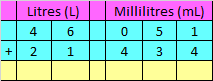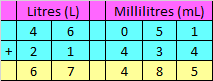Hence, the sum is 67 L 485 mL

Correct Answer – b) 67 L 485 mL

Q.6) Explanation – Measuring Capacity Worksheets

Step I: Write Litres (L) and Millilitres (mL) aligned in columns properly.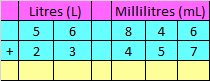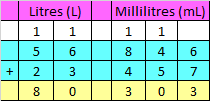Hence, the sum is 80 L 303 mL

Correct Answer – b) 80 L 303 mL

Q.7) Explanation – Measuring Capacity Worksheets

Step I: Write Litres (L) and Millilitres (mL) aligned in columns properly.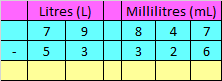Step II: Subtract Litres (L) and Millilitres (mL) as we subtract numbers in Subtraction.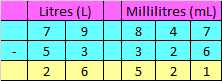Hence, the difference is 26 L 521 mL

Correct Answer – 26 L 521 mL

Q.8) Explanation – Measuring Capacity Worksheets

Step I: Write Litres (L) and Millilitres (mL) aligned in columns properly.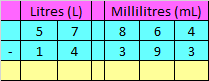Step II: Subtract Litres (L) and Millilitres (mL) as we subtract numbers in Subtraction.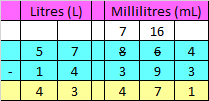Hence, the difference is 43 L 471 mL

Correct Answer – a) 43 L 471 mL